Next: Strategy and Planning Up: Vision Processing Previous: Symmetry Detection

## Color Model Classification

We have now localized a set of possible candidates for objects of interest (balls and pockets). To recognize and classify these objects, we propose the use of color models. We train a set of probabilistic color models for the pockets and the balls (cue ball, 8-ball, the seven striped balls and the seven solid balls). We also train models of other objects that might appear in the image as false alarms: a color model of the pool cue and the player's hand. This process is identical to the color modeling used for the table and we obtain a total of 20 models which have a form similar to Equation. We shall refer to these models as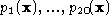.

Figureand Figureshow the color modeling process for the solid red ball and the striped orange ball. For each model, we begin with a distribution of pixels in RGB space as shown in Figure(a) and Figure(a). These sets of 3 dimensional RGB vectors are labeled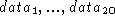. For each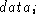we compute a model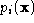when we first train the system.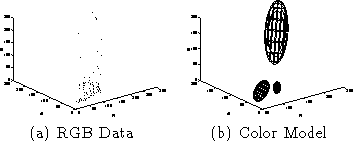Figure: The Solid Red Ball (Trained)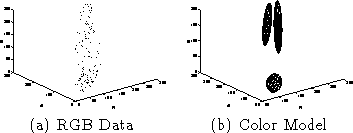Figure: The Striped Orange Ball (Trained)

Next we apply all 20 color models to examine each of the possible candidate symmetry peaks. Around each peak, we collect a small window of pixels. All non-table pixels (i.e. the ones that did not match the table's color model) are collected to form a distribution of RGB data similar to the one in Figure(a). This test distribution will be called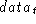. We form a single Gaussian color model (because EM with multiple Gaussians would be too slow to compute online). This model, shown in Figure(b), is called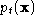.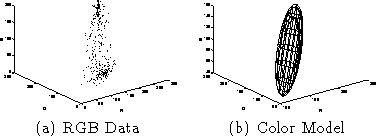Figure: The Test Object (Orange Striped Ball)

To classify our test distributionwe use a common distance metric between probabilistic models. This metric is the Kullback-Liebler divergence. By measuring the 'distance' between test dataand our training data,for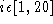we can see how similar it is to other objects. We determine the closest model i for each symmetry peak and label that peak accordingly. This process is iterated over all the symmetry peaks which are ultimately labeled as solid ball, striped ball, cue ball, 8-ball, pocket and 'other'.Next: Strategy and Planning Up: Vision Processing Previous: Symmetry Detection

Tony Jebara
Wed Feb 18 18:52:15 EST 1998